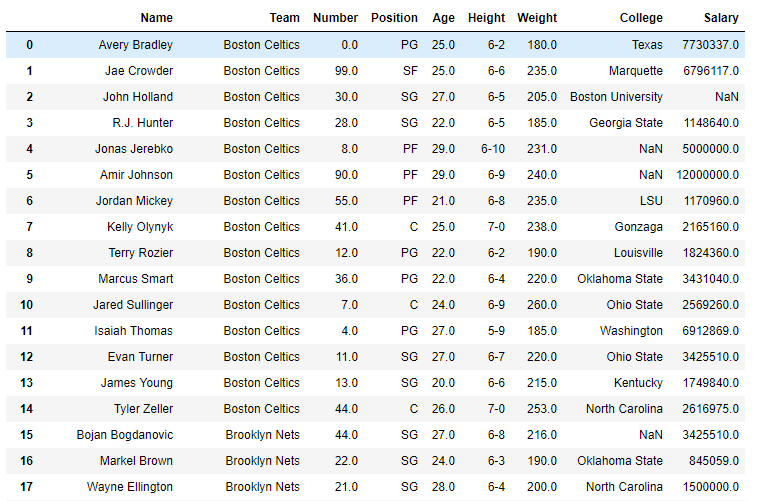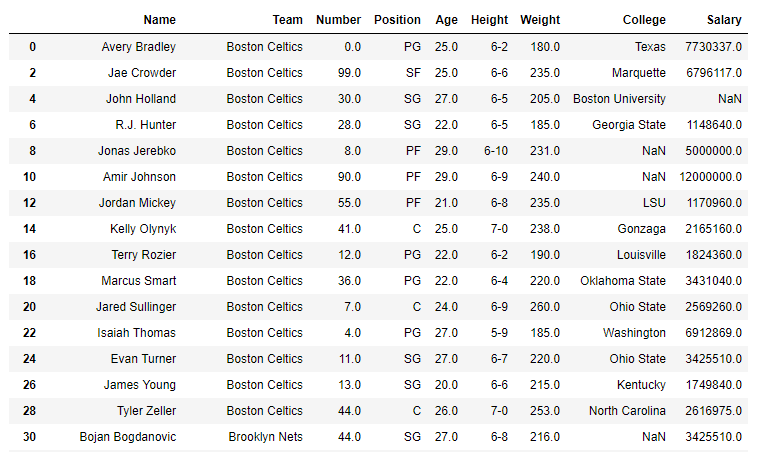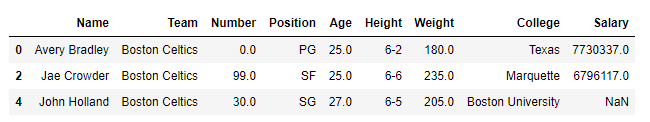# Python | Pandas dataframe.take()

• Last Updated : 23 Nov, 2018

Python is a great language for doing data analysis, primarily because of the fantastic ecosystem of data-centric python packages. Pandas is one of those packages and makes importing and analyzing data much easier.

Pandas` dataframe.take()` function return the elements in the given positional indices along an axis. This means that we are not indexing according to actual values in the index attribute of the object. We are indexing according to the actual position of the element in the object.

Syntax: DataFrame.take(indices, axis=0, convert=None, is_copy=True, **kwargs)

Parameters :
indices : An array of ints indicating which positions to take.
axis : The axis on which to select elements. 0 means that we are selecting rows, 1 means that we are selecting columns
convert : Whether to convert negative indices into positive ones. For example, -1 would map to the len(axis) – 1. The conversions are similar to the behavior of indexing a regular Python list.
is_copy : Whether to return a copy of the original object or not.
**kwargs : For compatibility with numpy.take(). Has no effect on the output.

Returns : An array-like containing the elements taken from the object.

Example #1: Use `take()` function to take some values over the index axis.

 `# importing pandas as pd``import` `pandas as pd`` ` `# Creating the dataframe ``df ``=` `pd.read_csv(``"nba.csv"``)`` ` `# Print the dataframe``df`Now we will modify the index labels for the demonstration purpose. Right now the labels are numbered from 0 to 914.

 `# double the value of index labels``df.index ``=` `df.index ``*` `2`` ` `# Print the modified dataframe``df`Let’s take the values at position 0, 1 and 2

 `# take values at input position over the index axis`` ` `df.take([``0``, ``1``, ``2``], axis ``=` `0``)`

Output :As we can see in the output, the values are selected based on the position but not on the index labels.

Example #2: Use `take()` function to take values at position 0, 1 and 2 over the column axis.

 `# importing pandas as pd``import` `pandas as pd`` ` `# Creating the dataframe ``df ``=` `pd.read_csv(``"nba.csv"``)`` ` `# Print the dataframe``df`Now we will take values at position 0, 1 and 2 over the column axis.

 `# take values over the column axis.`` ` `df.take([``0``, ``1``, ``2``], axis ``=` `1``)`

Output :My Personal Notes arrow_drop_up